ProfessorBurke.org

Where Mathematics, Education and Technology Meet

Generalized Parabolas - Powered by GeoGebra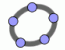A parabola is the locus of all points equidistant from a given point, the focus, and a line, the directrix. The September 2011 issue of The College Mathematics Journal (published by MAA) contains an article by Dan Joseph, Gregory Hartman and Caleb Gibson titled Generalized Parabolas (available online if a member/subscriber or through jstor: http://www.jstor.org/pss/10.4169/college.math.j.42.4.275 if you have access to jstor). In their article they investigate what happens if you change the directrix in the definition above to a general curve, for example, a parabola (see example 3 below). The authors took an analytical approach, using Mathematica to find the equation of each generalized parabola. I recognized GeoGebra could be used for a purely geometrical investigation. Examples 3 through 11 below reproduce examples from the paper sited above. The routine that produced the examples below is available for use here. Example 1 gives some instructions on using the generalized parabolas GeoGebra manupulative.
 Example 1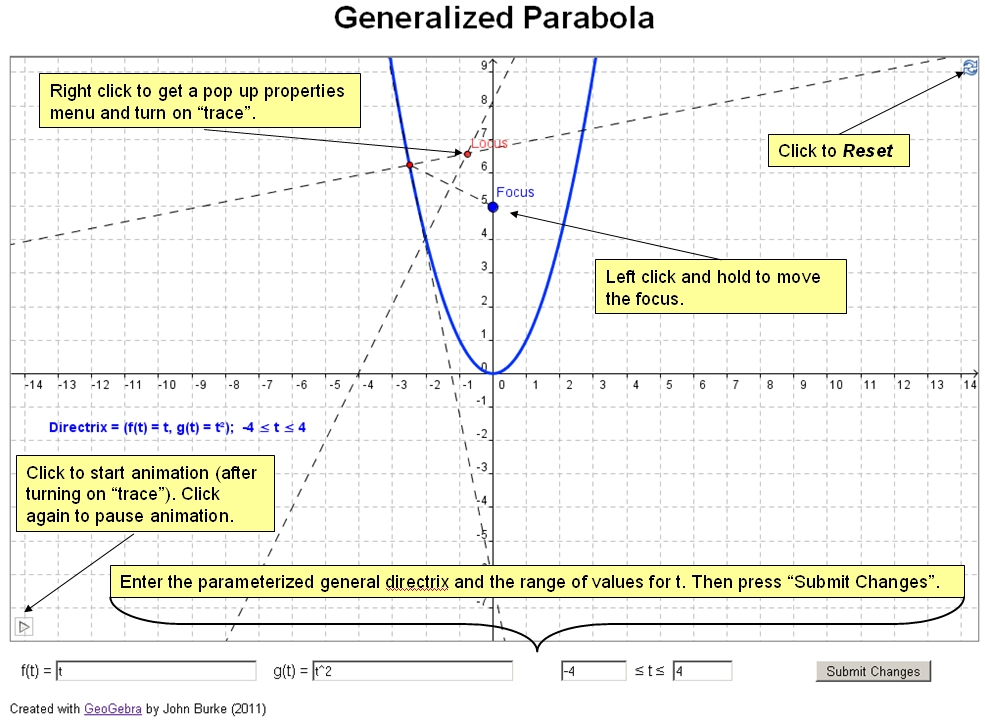How To Use

 Example 2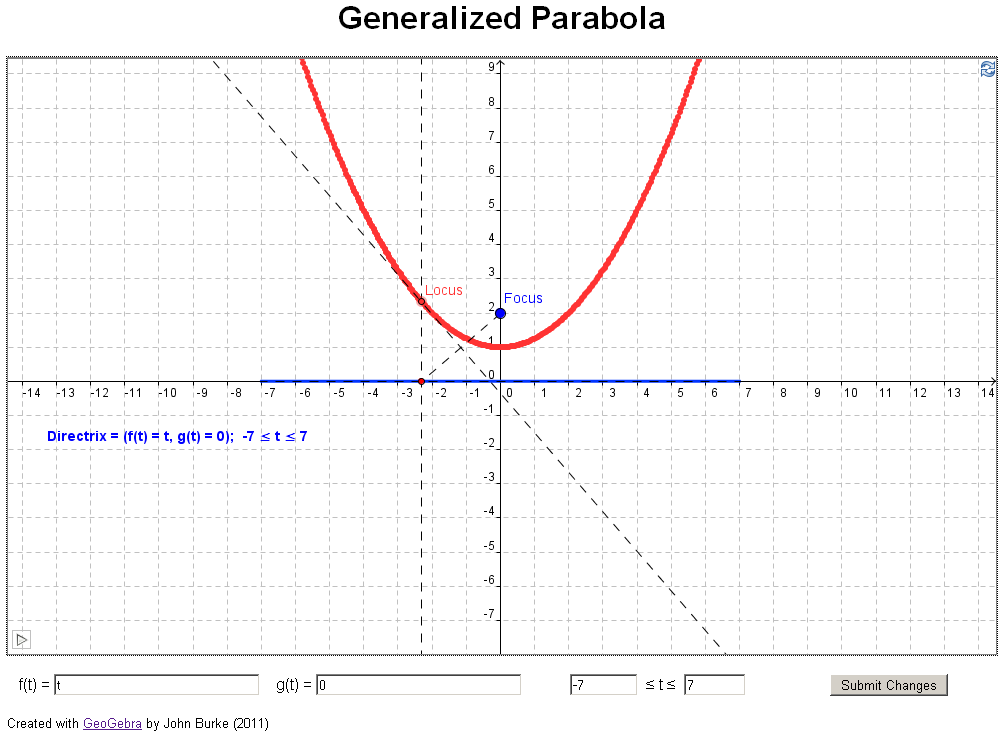Classic Parabola

 Example 3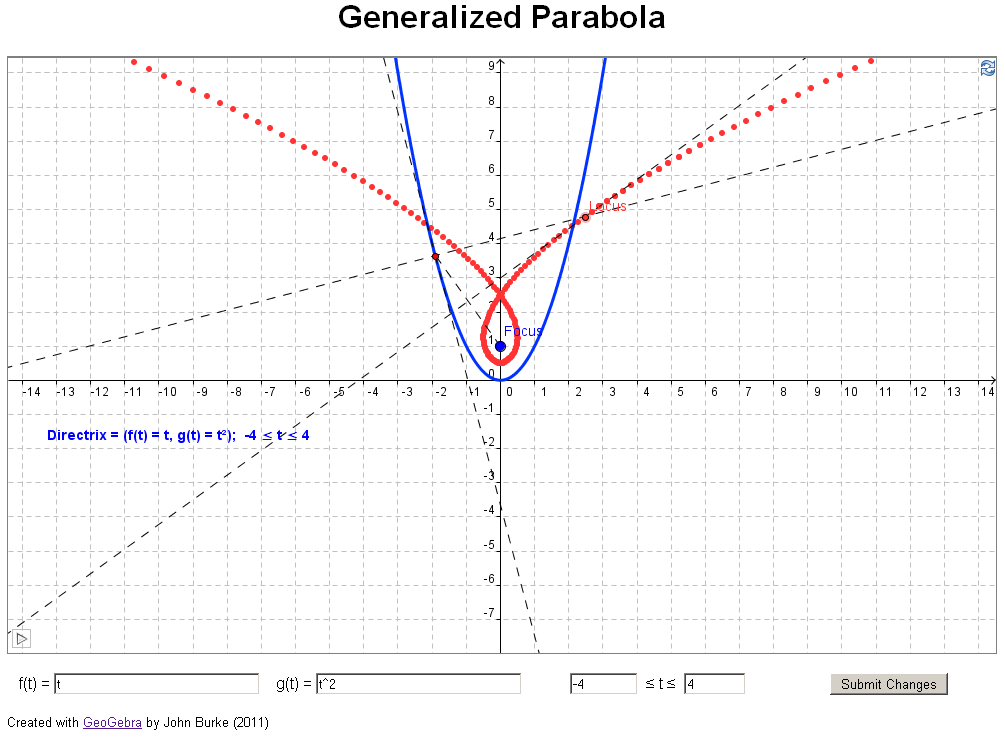Example 4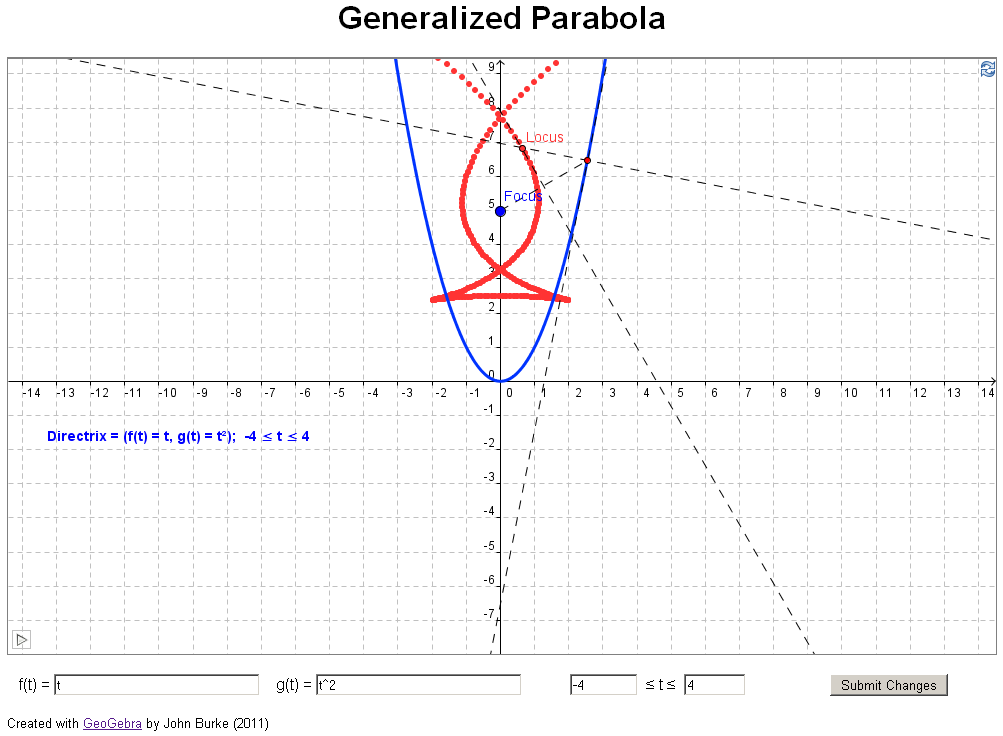Example 5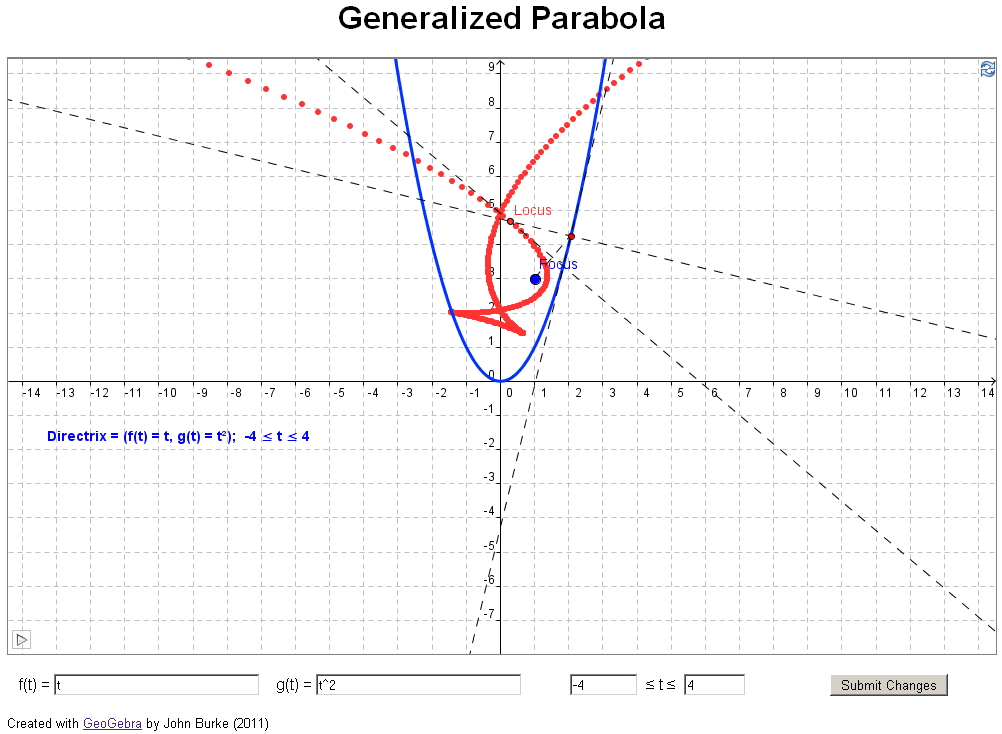Example 6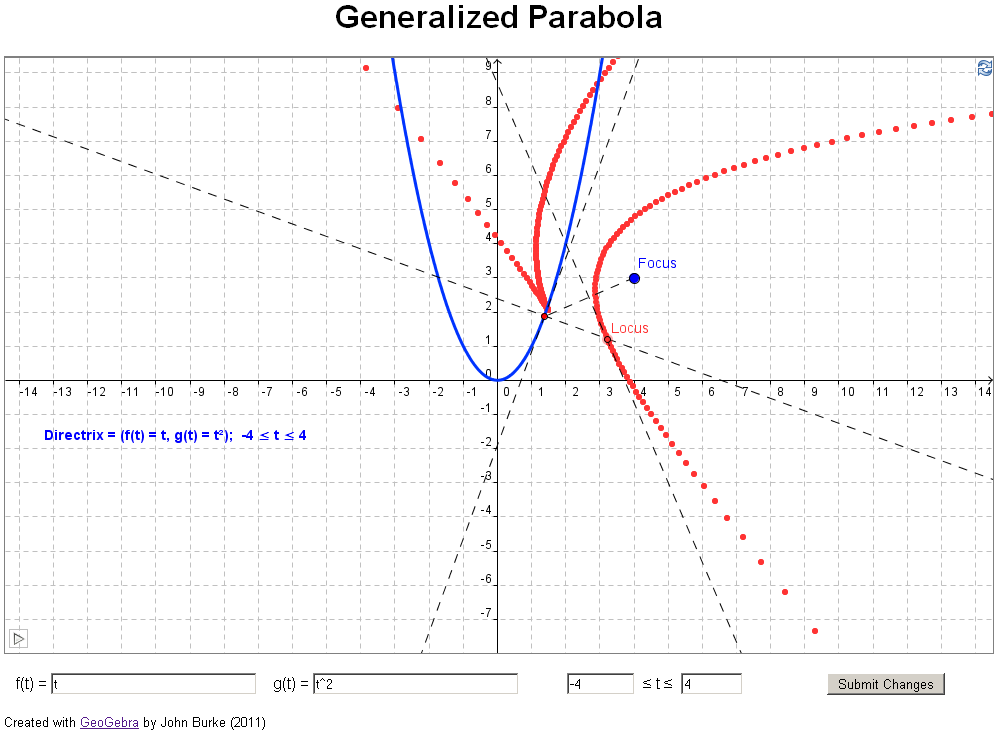Example 7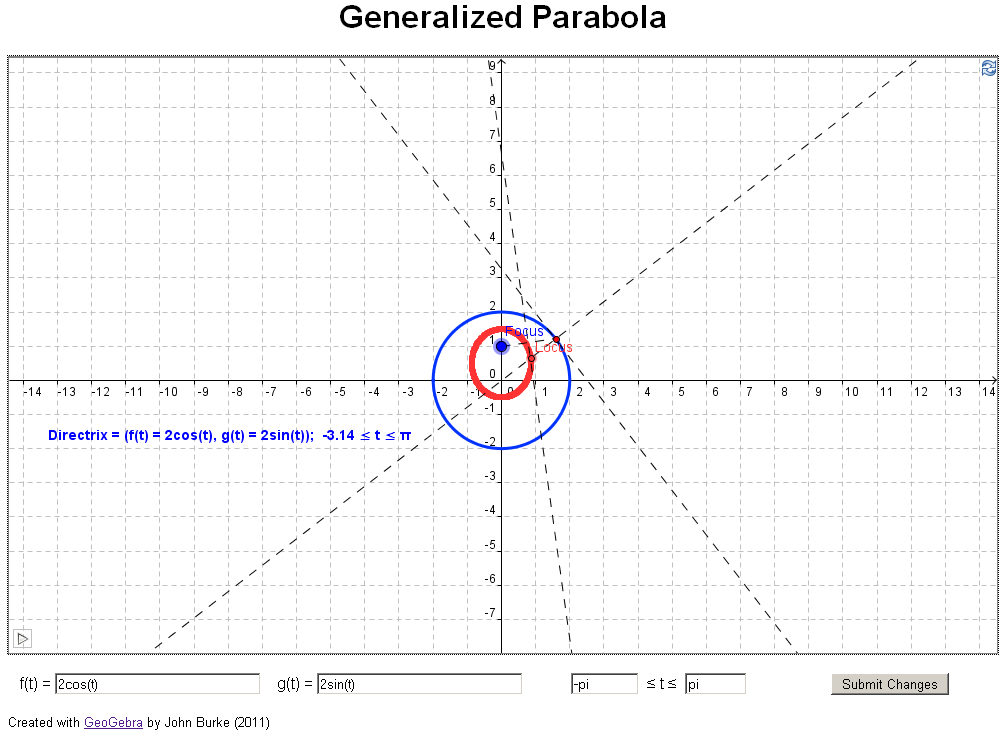Example 8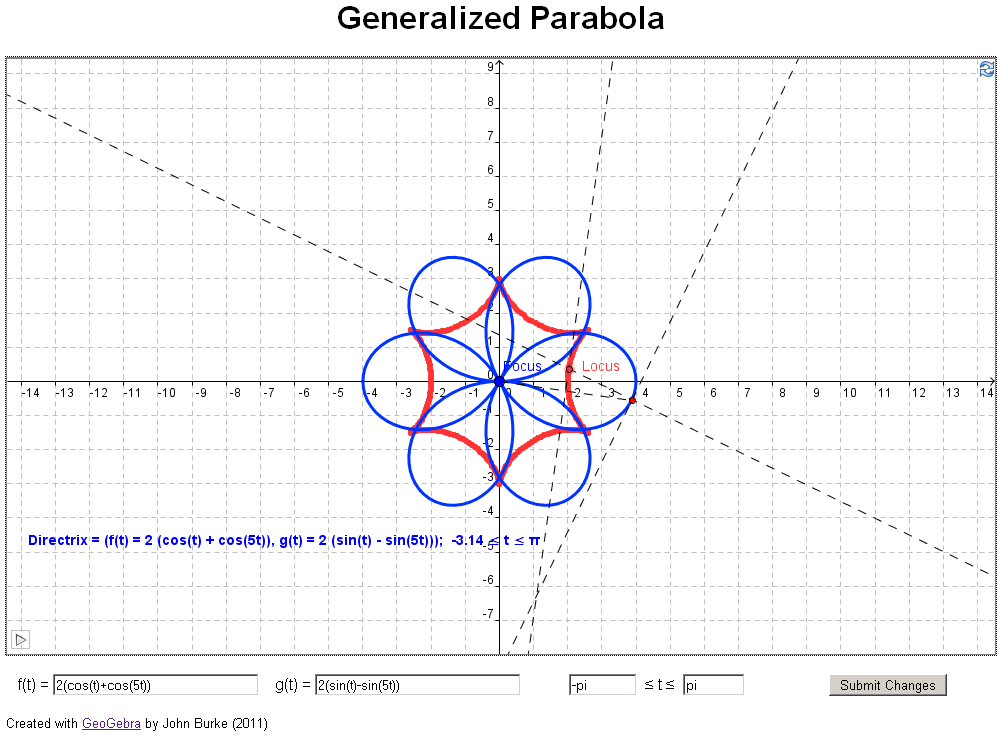Example 9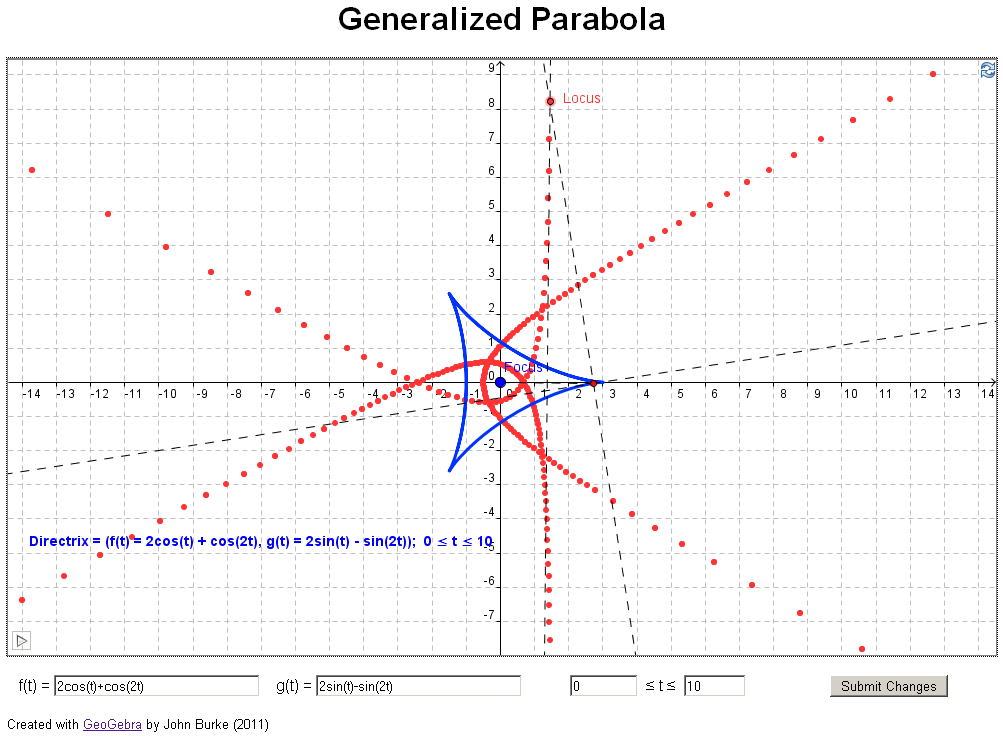Example 10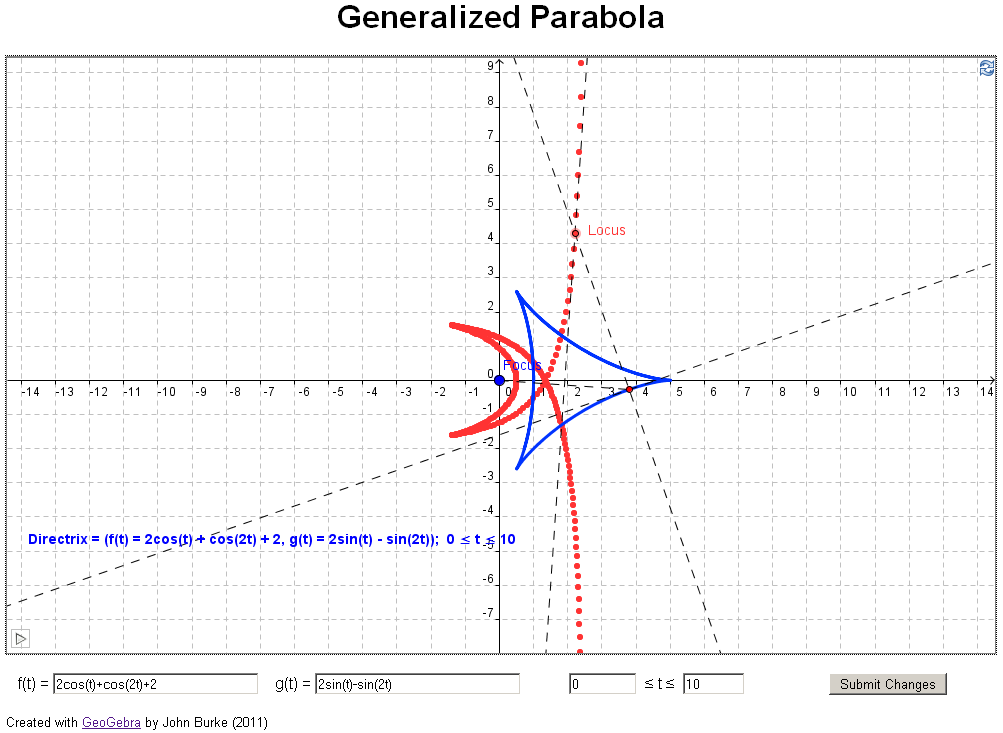Example 11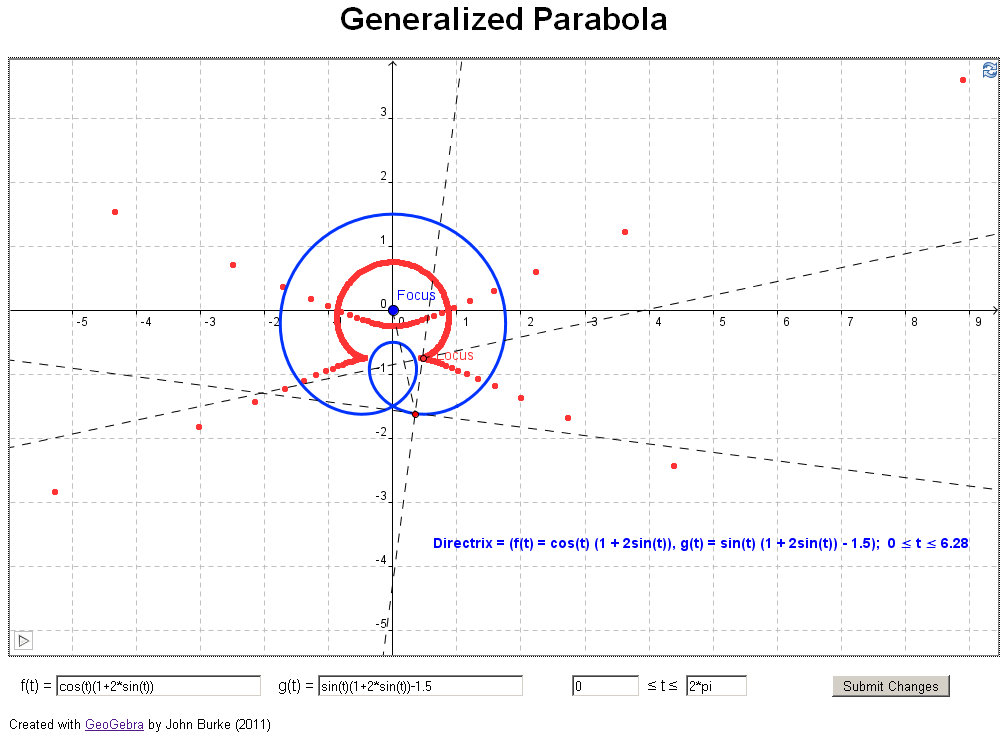Contact Me                                                                                                                                                      © 2007-2018 John Burke